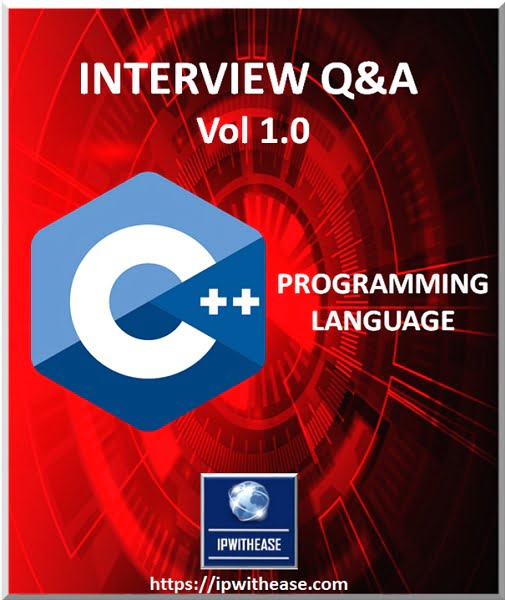# C++ Interview Questions

##ANSWERS @https://ipwithease.com/shop/

1. What is C++?
2. Differentiate declaration and definition?
3. Explain what is a class in C++?
4. How can you specify a class in C++?
5. What is the difference between realloc() and free()?
6. Define an inline function?
7. What is the difference between an ARRAY and a LIST?
8. What is a template?
9. What are the features of C++ different from C?
10. What are tokens in C++?
11. Define Constructors?
12. Define destructors?
13. Differentiate between Object and Instance?
14. What do you mean by reference variable in C++?
15. What are the basic elements of a function in C++?
16. Explain what is Loop function? What are different types of Loops?
17. Explain C++ exceptional handling?
18. Mention what are the types of Member Functions?
19. Explain what is multi-threading in C++?
20. Explain upcasting in C++?
21. Explain what is pre-processor in C++?
22. What is namespace std?
23. What is the difference between prefix and postfix versions of operator ++()?
24. What is public, protected and private?
25. What are the basic components of a class?
26. What do you mean by ‘void’ return type?
27. State the dissimilarity between delete and delete[]?
28. What is a Storage Class? Mention the Storage Classes in C++.
29. What do you mean by persistent and non-persistent objects?
30. What are the Comments in C++?
31. How do you define/declare constants in C++?
32. What is the difference between equal to (==) and Assignment Operator (=)?
33. What is a Reference Variable in C++?
34. What are the Extraction and Insertion operators in C++? Explain with examples.
35. Explain Pass by value and Pass by reference?
36. What is a Static Variable?
37. What is Structure?
38. What is Namespace?
39. Enlist the difference between Method Overloading and Method Overriding in C++?
40. List the various OOPs concepts in C++?
41. Define data abstraction in C++?
42. Define encapsulation?
43. Explain the main difference between a class and an object?
44. Define inheritance?
45. Define abstract class in C++?
46. Name the data type which can be used to store wide characters in C++.
47. Explain Inheritance in C++?
48. Give the full form of STL in C++?
49. When should we use multiple inheritance?
50. Give the difference between structures and class?